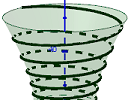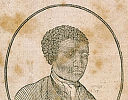# Posts tagged ‘Real-world math’

## Intmath Newsletter: Poly equations, charts, potential, water

1. New on IntMath: Equations graph solver
2. Resources: Charts
3. Math in the news: Lenses, integral equations
4. Math movies: Potential
5. Math puzzle: Age
6. Final thought: Water

## IntMath Newsletter: Calculators, atomic clocks, tensors

1. On IntMath: Calculators
2. Music lab, Webinars
3. AI, atomic clocks
4. Tensor, irrationals
5. Math puzzle
6. They knew

## IntMath Newsletter: CSS matrix, Humble Pi, van Gogh

1. New on IntMath: CSS matrix math
3. Math in the news: Proof
4. Math movies: Parker, van Gogh
5. Math puzzle: Mystery object
6. Final thought: Dry leaves

## IntMath Newsletter: Valentine’s, applets, maps

0. Geometry of love
1. New on IntMath
2. Quirky measurements
3. Resources: Map projections
4. Math movies:
5. Math puzzle
6. Final thought

## IntMath Newsletter: Hexagon, units, primes, Queen

1. New on IntMath: CSS Animated Hexagon Wave
2. Units
3. Math in the news: Almost prime numbers
4. Brian May - astrophysicist
4. Math movies: Data portraits and fingers
5. Math puzzle: Divisibility
6. Final thought: It's up to us

## Units: Calories and kilogramsHow many calories? and the way the kilogram was defined has been in place since 1799. It recently got a makeover.

## IntMath Newsletter: Model, animations, software

1. New on IntMath: EFFEKT bridge model, Lissajous animations
2. Resources: oPhysics, topol0gy software
3. Math in the news: 4-color graph problem
4. Math movies: Statistics, time
5. Math puzzle: Factorials
6. Final thought

## Spiral around a hyperboloid - the Effekt bridgeWe explore how to find the equations for a spiral around a hyperboloid.

## IntMath Newsletter: Series, clock, Simpsons

1. New on IntMath: Infinite series, Spinning clock
2. Resources: Simpsons math, Unknowables
3. Math in the news: Plimpton 322
4. Math movies
5. Math puzzle: EFFEKT
6. Final thought: Actions

## Math movie: The exceptional life of Benjamin BannekerSelf-taught mathematician and astronomer Benjamin Banneker excelled despite little formal education and prejudice.

## Full archive

See the blog sitemap for all previous articles.